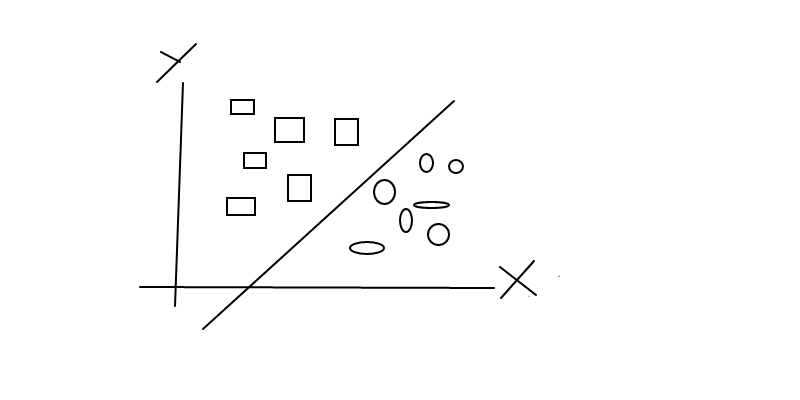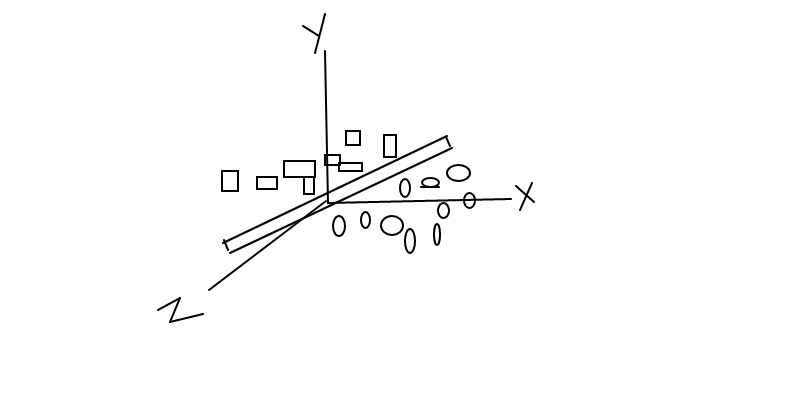# Why learn Linear Algebra ?

As you have already studied about linear algebra in your high school and college. Linear algebra is fundamental to almost every field of mathematics. And for the reminder, Linear algebra is the mathematical branch of linear equations such as Linear maps. And their representations by matrices and in vector spaces. In machine learning, linear algebra plays a vital role.

Linear algebra is a must-have prerequisite for data science and machine learning. If you want to build a strong data science career then linear algebra is a vital part of its base. You may wonder why you need to learn the linear algebra for learning machines. Let ‘s explore the need for a data science to get linear algebra.

Data is the crucial input for data science. Without data-there is no science which can be used to derive insights. Linear algebra is how the various models and algorithms are expressed in the data. These basic data expressing units-vectors and matrices-are the primary inputs on which further processing is carried out.The mathematical thinking behind those units helps us to better understand how the ML algorithms interpret and integrate data.

If you ever come across a beautiful picture or image, you really look at a matrix. On any program, when you do operations such as cropping, filtering, scaling or rotation of images, you actually do linear algebra operations in the backend. Linear algebra allows advanced operations like image compression too.And if you are interested in text as opposed to photos, the linear algebra still get around. Any piece of text must be transformed into a mathematical form before applying any ML algorithm-and most of the time we transform it into a matrix. In short, whether text or pictures, you have to enter through the matrix into the world of linear algebra.

Deep learning is the root (you know it!)-Linear Algebra again! They give you a better understanding about how algorithms actually operate under the hood, so you can make better decisions. Deep learning , computer vision, natural language processing, etc.-undoubtedly use linear algebra as their foundation. If you are trying to study data science and become an expert, mathematics paves the way with ample amounts of linear algebra.

Lets take a real world example . In Machine learning, we use linear algebra for a specific purpose with a specific problem. Lets start with a 2-Dimensional or as for simple say’s 2 features example. lets say we have one feature as a rectangle and another one as a circle. To separate this figure, we can draw a linear line where everything that belongs to one side of the line is a rectangle and another side is a circle.2-Dimensional linear algebra

And imagine for a 3-Dimensional spaces, Can we do the same thing for 3-D spaces as we did for 2-D spaces. What will be the figure be like with 3 features?3 Dimensional linear algebra

According to the figure above, we can say that everything on the one side of the plane is of one category as a rectangle and everything on the other side is another category as a circle. In this way we can plot the 3 Dimensional spaces or data points with 3 distinct features.

By learning from above 2D and 3D data points, we learned that , In 2-D we have a line & in 3-D, we have a plane.

So, we have learned about 2-D, and 3-D data points, what about 4-D,5-D,…, 10-D, 50-D, 100-D or n-D ?

As we know that it is not possible to visualize the data with above 4-D and so on. That’s the place where linear algebra shines. Linear algebra is a magnificent tool for understanding the n-D datasets. It provides a mathematical tool to operate with such big n-Dimensional spaces.

I post articles on Data Science | Machine Learning | Deep Learning . Connect with me on Linkedln: https://www.linkedin.com/in/bibek-shah-shankhar/

## More from Bibek Shah Shankhar

I post articles on Data Science | Machine Learning | Deep Learning . Connect with me on Linkedln: https://www.linkedin.com/in/bibek-shah-shankhar/